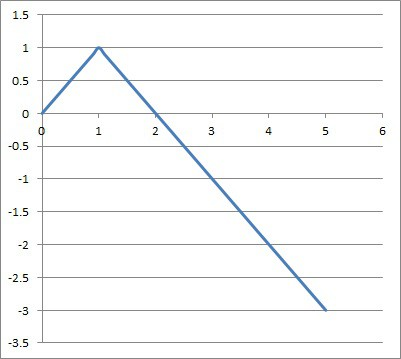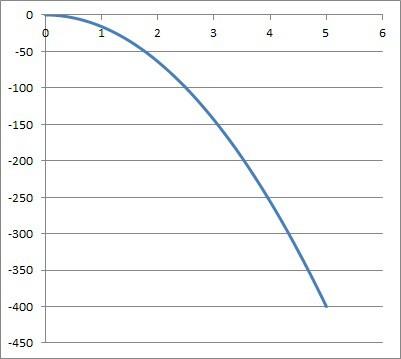# Can Displacement Be Negative? (What It Means & 3 Key Ideas)

Displacement is used in math and physics to describe the position of an object relative to a “zero” (starting) point.  However, it can be a little tricky to navigate the signs (positive and negative) of displacement as it relates to position.

So, can displacement be negative?  Displacement of an object can be negative, such as when the object falls below its “zero” (starting) height. An object that starts at zero with negative velocity will have negative displacement. Negative velocity means the displacement of an object is decreasing (moving in a negative direction).

Of course, negative displacement is not theoretical, since it occurs when an object falls below its starting height.

In this article, we’ll talk about negative displacement and when it can happen.  We’ll also look at some examples to make the concept clear.

Let’s get started.

## Can Displacement Be Negative?

Displacement can be negative in physics or calculus, such as when an object falls below its starting height (due to the effects of gravity).

Velocity is negative whenever position is decreasing.  In this case, an object’s displacement is decreasing, meaning it becomes closer to zero if positive, or becomes more negative if already negative.

It is important to note that negative velocity (or negative acceleration) does not always mean negative displacement.  However, given enough time, a negative velocity will eventually lead to negative displacement.

The table below helps to illustrate what happens with acceleration, velocity, and speed when they have different signs.

## What Does Negative Displacement Mean?

Negative displacement means an object is below its starting height (or behind or “to the left of” its starting position).  Negative displacement indicates one of the following:

• The object has moved below its starting height (or to the left of its starting position on a number line).
• The object has moved up and down (forward and backward), but has moved a greater distance down than up (or backward than forward).

Note that an object with negative displacement can be at rest (zero velocity), or it can be moving in a positive direction (positive velocity) or negative direction (negative velocity).

A negative displacement just means that the cumulative effect of the object’s movement puts it below its starting height (or behind its starting position).

An object with negative displacement can also have either positive or negative acceleration.

Remember:

• Displacement tells us where an object is, in relation to its “zero” (starting) position.
• Velocity tells us how fast an object is traveling, and in what direction.
• Acceleration tells us how fast an object is changing velocity.

## What Is The Difference Between Positive & Negative Displacement?

The difference between positive and negative displacement is the position of the object relative to the zero or starting point:

• Positive displacement means that the object is above its starting height, or ahead of its starting position.
• Negative displacement means that the object is below its starting height, or behind its starting position.

Displacement does not tell us where an object has been.  Instead, it tells us where the object is now, at a particular time, relative to where it started from (the zero position).

## Negative Displacement Graph

The graph below shows the displacement of an object over time:Displacement (on the y axis) is positive from t = 0 to t = 2 (on the vertical axis). Displacement is negative from t = 2 to t = 5. Displacement is zero at t = 0 and t = 2.

When the graph is above the x-axis, the displacement is positive, meaning that the object is above its starting height.  For example, at time t = 0 to time t = 2, the object is above its starting height.

Note that the peak of the graph (at time t = 1) marks the farthest above the starting point that the object gets in the first 5 seconds (1 unit above the starting point).

When the graph is below the x-axis, the displacement is negative, meaning that the object is above its starting height.  For example, at time t = 2 to time t = 5, the object is below its starting height.

Note that the bottom of the graph (at time t = 5) marks the farthest below the starting point that the object gets in the first 5 seconds (3 unit below the starting point).

When the graph touches the x-axis, the object is at a height of zero (the same as its starting height). This happens at time t = 0 and time t = 2.

## Example Of Negative Displacement

One of the most common examples of negative displacement is an object falling due to the force of gravity.

If you drop an object from rest, it will fall towards the ground due to gravity.  If the starting position is the “zero” height, then the object’s height becomes more negative as it falls.

You can see a graph of the negative displacement of a falling object below.The displacement of a falling object due to gravity starts at zero and becomes more negative as time passes. Note that the object falls faster as time passes, since the acceleration due to gravity has a cumulative effect on the velocity.

## Conclusion

Now you know more about negative displacement, what it means, and how it can happen.  You also know how velocity relates to displacement.